• ### Having lots of switches into Pd

I would suggest to not mix the analog values with the digital ones. The first code could be changed to the following (using Serial.print() with the [serial_print] abstraction):

``````void setup()
{
for(int i = 2; i < 14; i++)
pinMode(i, INPUT);
Serial.begin(115200); // perhaps use a faster baud rate
}

void loop()
{
Serial.print("knobs"); // use "knobs" as a keyword so you can receive
// the knob values as a list with a [r knobs] in Pd
for(int i = 0; i < 6; i++){
unsigned int knob = analogRead (i);
Serial.print(" "); // first print a white space to separate the "knob" keyword from the values
// and the values from each other
Serial.print(knob); // then  print the actual knob value
}
Serial.println(); // finally print a newline character to denote end of data for keyword "knobs"

// the same technique applies to the switches too
// receive the switch values as a list with [r switches]
Serial.print("switches");
for(int i = 2; i < 14; i++) {
Serial.print(" ");
Serial.print(switchVal);
}
Serial.println();
}
``````

As for writing to several outputs you need to set which output you want to write to and then the value you want to write. Here's an example that writes to several different digital outputs:

``````int pin = 0;
int val = 0;
// some random pins
int pins = {3, 4, 5, 6];

void setup() {
for (int i = 0; i < 4; i++) {
pinMode(pins[i], OUTPUT);
digitalWrite(pins[i], LOW);
}

Serial.begin(115200);
}

void loop() {
if (Serial.available()) {
static int temp;
if (isDigit(in)) {
temp = temp * 10 + in - '0';
}
else if (in == 'p') {
pin = temp;
temp = 0;
}
else if (in == 'v')  {
val = temp;
temp = 0;
digitalWrite(pins[pin], val);
}
}
``````

With the code above you can send messages like this one `print \$1p\$2v` in Pd to the [comport] object. \$1 is the number of the pin you want to light up starting from 0 and incrementing by 1 (so the first pin used which is pin 3 in the Arduino code would be 0 in the Pd patch), and \$2 is the value, 0 or 1.
Note though that in the first code (and the code you posted), you're using all digital pins as inputs so there's no pin left to use as output. If you want to combine these two chunks of code you'll have to use less pins as inputs and leave some to be used as outputs.

• @alexandros thanks, it all works!

I merged the codes for in and outs and also changed so you can set up all of the pins with arrays (as you did in the latter code). Then you can set up the pins in any order.

Here is the Arduino code. Do you spot any errors I may have introduced?

``````//number of elements in array need to match for() cycles
int pinsIn = {2, 7, 10, 11};
int pinsAnalog = {0, 2, 3};

int pin = 0;
int val = 0;
// some random pins
int pinsOut = {3, 4, 5, 6};

void setup()
{
//set up a total of pins for input (has to match number of elements in array)
for(int i = 0; i < 4; i++)
pinMode(pinsIn[i], INPUT);

for (int i = 0; i < 4; i++) {
pinMode(pinsOut[i], OUTPUT);
digitalWrite(pinsOut[i], LOW);
}

Serial.begin(115200); // perhaps use a faster baud rate
}

void loop()
{
Serial.print("knobs"); // use "knobs" as a keyword so you can receive
// the knob values as a list with a [r knobs] in Pd
for(int i = 0; i < 3; i++){
unsigned int knob = analogRead (pinsAnalog[i]);
Serial.print(" "); // first print a white space to separate the "knob" keyword from the values
// and the values from each other
Serial.print(knob); // then  print the actual knob value
}
Serial.println(); // finally print a newline character to denote end of data for keyword "knobs"

// the same technique applies to the switches too
// receive the switch values as a list with [r switches]
Serial.print("switches");
for(int i = 0; i < 4; i++) {
Serial.print(" ");
Serial.print(switchVal);
}
Serial.println();

//handle digital outputs
if (Serial.available()) {
static int temp;
if (isDigit(in)) {
temp = temp * 10 + in - '0';
}
else if (in == 'p') {
pin = temp;
temp = 0;
}
else if (in == 'v')  {
val = temp;
temp = 0;
digitalWrite(pinsOut[pin], val);
}
}
}
``````

In Pd I was not able to use [r switches] or [r knobs] but had to use [route]. Is this the correct way to use [serial_print]?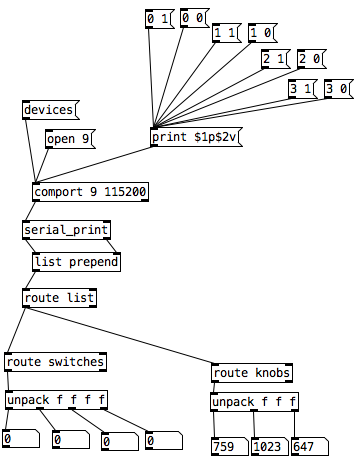And here it is on the breadboard: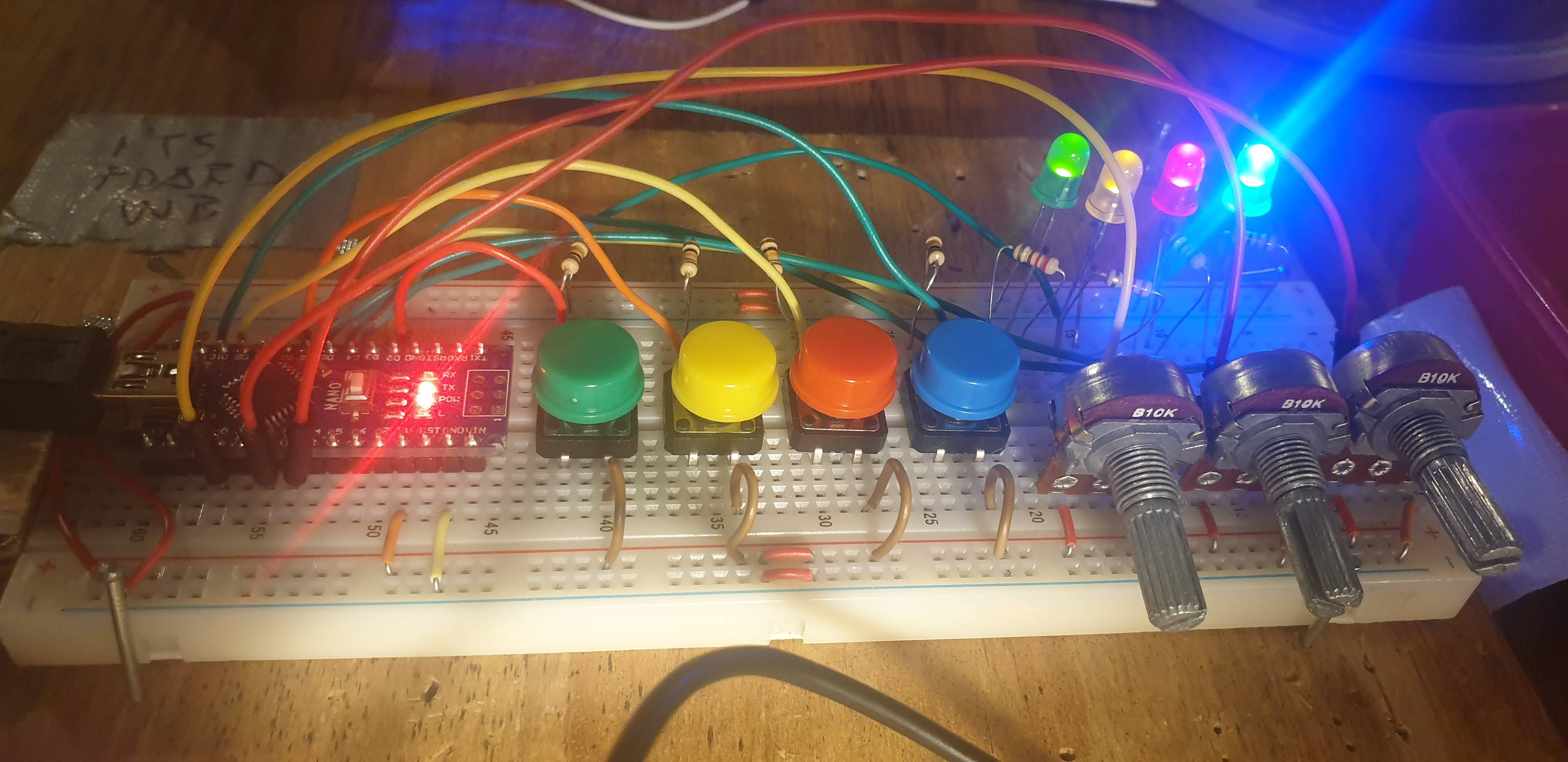• It looks alright. Perhaps you're facing the same issue with me and the Arduino prints 10 when you try to print 13. Read the README file of the GitHub repository where I explain this and how to check it. Maybe you need [serial_print13] instead of [serial_print]. [receive] objects should normally work, that's why I suggest the above.

Analog values tend to change all the time due to noise (unless you use the ResponsiveAnalogRead library which I highly recommend). Digital values though are much cleaner. For these values you can also do a test and see if a value has changed and print it only in that case. You'll need a variable for each button to hold its previous value and test against the new value. If they are different then print and save the new value to the variable for the next test.
This way you should print each button separately and not with a loop. Hope this all makes sense.

• @alexandros

Hi, I am trying to add the code for pwm control (from tutorial 5) to the code above but I can not get it to work. Could you help me out plz? I would like to use 6 pwm outputs so I figure it should be set up as the other digital outputs.

• @cfry can you share your code and patch and tell us what is not working?

• @alexandros
This code sort of works with wip_multiple_PWM.pd

``````// merging works but pwm leds are choppy.

// number of elements in arrays need to
// match for() cycles in void setup and void loop
int pinsIn = {2, 4};
int pinsAnalog = {0, 1, 2, 3, 4, 5, 6, 7};

int pin = 0;
int val = 0;
int pinsOut = {7, 12};

//TMP setup pwm:

// variables to hold pin numbers
int pwmLED1 = 3;
int pwmLED2 = 5;
int pwmLED3 = 6;
int pwmLED4 = 9;
int pwmLED5 = 10;
int pwmLED6 = 11;

// variables to hold pin states
int pwmLEDvalue1;
int pwmLEDvalue2;
int pwmLEDvalue3;
int pwmLEDvalue4;
int pwmLEDvalue5;
int pwmLEDvalue6;

//should this be omitted and use the a
// variable to hold and assemble incoming data
int temporary;

//END TMP pwm setup

void setup()
{
//set up a total of pins for digital input (has to match number of elements in array)
for(int i = 0; i < 2; i++)
pinMode(pinsIn[i], INPUT);

for (int i = 0; i < 2; i++) {
pinMode(pinsOut[i], OUTPUT);
digitalWrite(pinsOut[i], LOW);
}

//DEFAULT works with thermistors,
//INTERNAL with transitor thermostats

analogReference(DEFAULT);

pinMode(A0, INPUT_PULLUP);
pinMode(A1, INPUT_PULLUP);
pinMode(A2, INPUT_PULLUP);
pinMode(A3, INPUT_PULLUP);
pinMode(A4, INPUT_PULLUP);
pinMode(A5, INPUT_PULLUP);
pinMode(A6, INPUT);
pinMode(A7, INPUT);

//TMP test pwm setup:

pinMode(pwmLED1, OUTPUT);
pinMode(pwmLED2, OUTPUT);
pinMode(pwmLED3, OUTPUT);
pinMode(pwmLED4, OUTPUT);
pinMode(pwmLED5, OUTPUT);
pinMode(pwmLED6, OUTPUT);

Serial.begin(115200); // perhaps use a faster baud rate
}

void loop()
{
Serial.print("knobs"); // use "knobs" as a keyword so you can receive
// the knob values as a list with a [r knobs] in Pd
for(int i = 0; i < 8; i++){
unsigned int knob = analogRead (pinsAnalog[i]);
Serial.print(" "); // first print a white space to separate the "knob" keyword from the values
// and the values from each other
Serial.print(knob); // then  print the actual knob value
}
Serial.println(); // finally print a newline character to denote end of data for keyword "knobs"

// the same technique applies to the switches too
// receive the switch values as a list with [r switches]
Serial.print("switches");
for(int i = 0; i < 2; i++) {
Serial.print(" ");
Serial.print(switchVal);
}
Serial.println();

//handle digital outputs
if (Serial.available()) {
static int temp;
if (isDigit(in)) {
temp = temp * 10 + in - '0';
}
else if (in == 'p') {
pin = temp;
temp = 0;
}
else if (in == 'v')  {
val = temp;
temp = 0;
digitalWrite(pinsOut[pin], val);
}
}

//TMP merge test PWMs:
while(Serial.available()){
if((inByte >= '0') && (inByte <= '9'))
temporary = 10 * temporary + inByte - '0';
else{
if(inByte == 'p'){
pwmLEDvalue1 = temporary;
temporary = 0;
}
else if(inByte == 'q'){
pwmLEDvalue2 = temporary;
temporary = 0;
}
else if(inByte == 'r'){
pwmLEDvalue3 = temporary;
temporary = 0;
}
else if(inByte == 's'){
pwmLEDvalue4 = temporary;
temporary = 0;
}
else if(inByte == 't'){
pwmLEDvalue5 = temporary;
temporary = 0;
}
else if(inByte == 'u'){
pwmLEDvalue6 = temporary;
temporary = 0;
}
}
analogWrite(pwmLED1, pwmLEDvalue1);
analogWrite(pwmLED2, pwmLEDvalue2);
analogWrite(pwmLED3, pwmLEDvalue3);
analogWrite(pwmLED4, pwmLEDvalue4);
analogWrite(pwmLED5, pwmLEDvalue5);
analogWrite(pwmLED6, pwmLEDvalue6);
//digitalWrite(dspLED, dspLEDstate);
}
}
``````

This is the code without PWM control. It works fine.

``````

//number of elements in arrays need to match for() cycles in void setup
int pinsIn = {6, 7, 8, 9};
int pinsAnalog = {0, 1, 2, 3, 4, 5, 6, 7};

int pin = 0;
int val = 0;
int pinsOut = {2, 3, 4, 5};

void setup()
{
//set up a total of pins for digital input (has to match number of elements in array)
for(int i = 0; i < 4; i++)
pinMode(pinsIn[i], INPUT);

for (int i = 0; i < 4; i++) {
pinMode(pinsOut[i], OUTPUT);
digitalWrite(pinsOut[i], LOW);
}
//DEFAULT works with thermistors,
//INTERNAL with transitor thermostats
// ELLER var det tvartom???
analogReference(DEFAULT);

pinMode(A0, INPUT_PULLUP);
pinMode(A1, INPUT_PULLUP);
pinMode(A2, INPUT_PULLUP);
pinMode(A3, INPUT_PULLUP);
pinMode(A4, INPUT_PULLUP);
pinMode(A5, INPUT_PULLUP);
pinMode(A6, INPUT);
pinMode(A7, INPUT);

Serial.begin(115200); // perhaps use a faster baud rate
}

void loop()
{
Serial.print("knobs"); // use "knobs" as a keyword so you can receive
// the knob values as a list with a [r knobs] in Pd
for(int i = 0; i < 8; i++){
unsigned int knob = analogRead (pinsAnalog[i]);
Serial.print(" "); // first print a white space to separate the "knob" keyword from the values
// and the values from each other
Serial.print(knob); // then  print the actual knob value
}
Serial.println(); // finally print a newline character to denote end of data for keyword "knobs"

// the same technique applies to the switches too
// receive the switch values as a list with [r switches]
Serial.print("switches");
for(int i = 0; i < 4; i++) {
Serial.print(" ");
Serial.print(switchVal);
}
Serial.println();

//handle digital outputs
if (Serial.available()) {
static int temp;
if (isDigit(in)) {
temp = temp * 10 + in - '0';
}
else if (in == 'p') {
pin = temp;
temp = 0;
}
else if (in == 'v')  {
val = temp;
temp = 0;
digitalWrite(pinsOut[pin], val);
}
}
}
``````

and here is the code from tutorial5 from Arduino for Pd'ers. It goes with arduinoforpdrs_tut5.pd

``````// variables to hold pin numbers
int pwmLED = 9;
int dspLED = 2;
// variables to hold pin states
int pwmLEDvalue;
int dspLEDstate;
//variable to hold and assemble incoming data
int temporary;

void setup()
{
pinMode(pwmLED, OUTPUT);
pinMode(dspLED, OUTPUT);
Serial.begin(9600);
}

void loop()
{
while(Serial.available()){
if((inByte >= '0') && (inByte <= '9'))
temporary = 10 * temporary + inByte - '0';
else{
if(inByte == 'p'){
pwmLEDvalue = temporary;
temporary = 0;
}
else if(inByte == 'd'){
dspLEDstate = temporary;
temporary = 0;
}
}
analogWrite(pwmLED, pwmLEDvalue);
digitalWrite(dspLED, dspLEDstate);
}
}
``````

I am aiming at using same type of array handling as for the digital outs.

Thanks a lot

• First of all, don't separate functions for reading the serial data, even if you want to read data for different kinds of stuff.

Combining digital with PWM pins should be sort of straight forward. Here's some example code:

``````int digitalPins = {2, 4, 7, 10};
// use an array for all the pins of similar type, e.g. PWM pins, like below
int pwmPins = {3, 5, 6, 9, 11, 12}; // can't remember the exact pin numbers with PWM, change accordingly

// a global variable to hold which LED we want to control (either digital or PWM)
int channel = 0;

void setup() {
for (int i = 0; i < 4; i++) {
pinMode(digitalPins[i], OUTPUT);
pinMode(pwmPins[i], OUTPUT);
Serial.begin(115200);
}

void loop() {
if (Serial.available()) {
static int temp;
if (isDigit(in)) temp = temp * 10 + in - '0';
else if (in == 'c') {
channel = temp;
temp = 0;
}
else if (in == 'd') {
digitalWrite(digitalPins[channel], temp);
temp = 0;
}
else if (in == 'a') {
analogWrite(pwmPins[channel], temp);
temp = 0;
}
}
``````

Check this code with Pd by sending messages of the type `print \$1c\$2d` for digital values and `print \$1c\$2a` for analog values. \$1 is the led number (incrementing, starting from 0), and \$2 is the value you want to write, 0-1 for digital and 0-255 for PWM.

• @alexandros now pwm and digital output work fine, but when I try to merge with digital and analog inputs it goes wrong. the leds flicker randomly. If you remove the code below
`// do input pins:` flicker stops. :/

``````int outPins = {2, 4, 7};

int pwmPins = {3, 5, 6, 9, 10, 11};

// a global variable to hold which LED we want to control (either digital or PWM)
int channel = 0;

int inPins = {12, 13};

int analogPins = {0, 1, 2, 3, 4, 5, 6, 7};

void setup() {

for (int i = 0; i < 3; i++) {
pinMode(outPins[i], OUTPUT);
}
for (int i = 0; i < 6; i++) {
pinMode(pwmPins[i], OUTPUT);
}
for(int i = 0; i < 2; i++) {
pinMode(inPins[i], INPUT);
}

pinMode(A0, INPUT_PULLUP);
pinMode(A1, INPUT_PULLUP);
pinMode(A2, INPUT_PULLUP);
pinMode(A3, INPUT_PULLUP);
pinMode(A4, INPUT_PULLUP);
pinMode(A5, INPUT_PULLUP);
pinMode(A6, INPUT);
pinMode(A7, INPUT);

//DEFAULT works with thermistors,
//INTERNAL with transitor thermostats
analogReference(DEFAULT);

Serial.begin(115200);

}

void loop() {

//do output pins:

if (Serial.available()) {
static int temp;
if (isDigit(in)) temp = temp * 10 + in - '0';
else if (in == 'c') {
channel = temp;
temp = 0;
}
else if (in == 'd') {
digitalWrite(outPins[channel], temp);
temp = 0;
}
else if (in == 'p') {
analogWrite(pwmPins[channel], temp);
temp = 0;
}
}

// do input pins:

Serial.print("analog"); // use "knobs" as a keyword so you can receive
// the knob values as a list with a [r analog] in Pd
for(int i = 0; i < 8; i++){
unsigned int analogVal = analogRead (analogPins[i]);
Serial.print(" "); // first print a white space to separate the "knob" keyword from the values
// and the values from each other
Serial.print(analogVal); // then  print the actual knob value
}
Serial.println(); // finally print a newline character to denote end of data for keyword "knobs"

Serial.print("digital");
for(int i = 0; i < 2; i++) {
Serial.print(" ");
Serial.print(digitalVal);
}
Serial.println();
}
``````
• @cfry why do you call pinMode on pins A0 through A7? You don't need to declare the analog pins. Is this for potentiometers? Is there a reason you need the input_pullup resistors on these pins?
What about the input dogotal pins?Are you using resistors in your circuit? Cause you're not using the input_pullups there.
Can you share a schematic of your circuit?

• I am at the moment experimenting with different setups and circuits using heat sensitive sensors and haptic feedback (and audio).

But what I am after is to be able to choose freely whatever combination of pin and pin configuration to be used with pd, when experimenting. So there is no specific circuit. I have several different scenarios that I want to try.

The actual pin settings and modes are not important, I just hope to be able to handle digital i/o and analog i/o at the same time.

I dont know how to debug in this setup either.

Thanks for helping@alexandros said:

@cfry why do you call pinMode on pins A0 through A7? You don't need to declare the analog pins. Is this for potentiometers? Is there a reason you need the input_pullup resistors on these pins?
What about the input dogotal pins?Are you using resistors in your circuit? Cause you're not using the input_pullups there.
Can you share a schematic of your circuit?

• @alexandros

...or am I trying to use the arduino with pd in a not suitable way? Would it be better to skip on the idea of using ins and outs at the same time and instead use two (nanos), one for ins and the other for outs?

• @cfry definitely not necessary to use two Arduinos. I can't really understand where your problem lies. You didn't reply to my earlier question about the pinMode calls where you use INPUT_PULLUP on analog inputs. Is this really necessary? Also, why not use the analog pins in an array like with the digital pins? It will save a lot of writing and it's less error prone.

One thing you might want to consider is having the Arduino notify Pd when it's done sending all its data and that it's ready to receive. That could be done at the end of the loop() function like this:

``````Serial.print("done ");
Serial.println("bang");
``````

And in Pd have a [r done] object which will output a bang. Use this bang to send data from Pd to the Arduino by unpacking a list with all the accumulated data, possibly with a [list-abs/list-drip].
I use this notification technique in my 3dPdModular system, but there's a LOT of data there and it was only necessary. Perhaps you could try it out.

• Thank you for replying and sorry if I am unclear. I will try to slow down a bit.

@alexandros said:

@cfry definitely not necessary to use two Arduinos. I can't really understand where your problem lies.

-The problem is that it does not work when I try to use an arbitrary combination of pins in different modes (Digital in, Digital out, Analog in, Analog out).

Another problem is that I have a hard time reading the code parts that are handling the conversion data in order to do the serial communication, although I understand the principle of it.

-I assumed that the problem lied in void ()loop as a conflict between the output and input handling sections. And that pinMode calls in void setup() was not relevant in this case. That is why I did not answer that question directly.

Also, why not use the analog pins in an array like with the digital pins? It will save a lot of writing and it's less error prone.

-I was foreseeing that I may want to configure those pins individually, but I can set it up with a loop for now.

One thing you might want to consider is having the Arduino notify Pd when it's done sending all its data and that it's ready to receive. That could be done at the end of the loop() function like this:

``````Serial.print("done ");
Serial.println("bang");
``````

And in Pd have a [r done] object which will output a bang. Use this bang to send data from Pd to the Arduino by unpacking a list with all the accumulated data, possibly with a [list-abs/list-drip].
I use this notification technique in my 3dPdModular system, but there's a LOT of data there and it was only necessary. Perhaps you could try it out.

-I will try that.

Since I consider this to be basically your code I assumed that you could easily just insert the code for both input and output in void loop() in a way that works and that would show me how to solve it. Then I would be able to do further explorations and it would be end of story.

I will digest this a bit and may start a new thread, since this one has slided from "Having lots of switches into Pd" to "having a working code template for communicating between Arduino and Pd using and an arbitrary mixture of pin modes".

Please let me know if this still does not make sense. Thank you so much!

• @cfry the thing is that the last bit of code you posted seems just fine, excluding the calling to pinMode where you're enabling the integrated pull-up resistors. Can you try that code removing these lines in setup(), and instead of heat sensitive sensors or whatever you're currently using, use simple potentiometers and verify whether that works or not? In case it doesn't work, please post a diagram of your circuit in some way (even hand-drawn is ok), as well as the full Arduino sketch and a screenshot of the Pd patch.

• @alexandros said:

@cfry the thing is that the last bit of code you posted seems just fine, excluding the calling to pinMode where you're enabling the integrated pull-up resistors. Can you try that code removing these lines in setup(), and instead of heat sensitive sensors or whatever you're currently using, use simple potentiometers and verify whether that works or not? In case it doesn't work, please post a diagram of your circuit in some way (even hand-drawn is ok), as well as the full Arduino sketch and a screenshot of the Pd patch.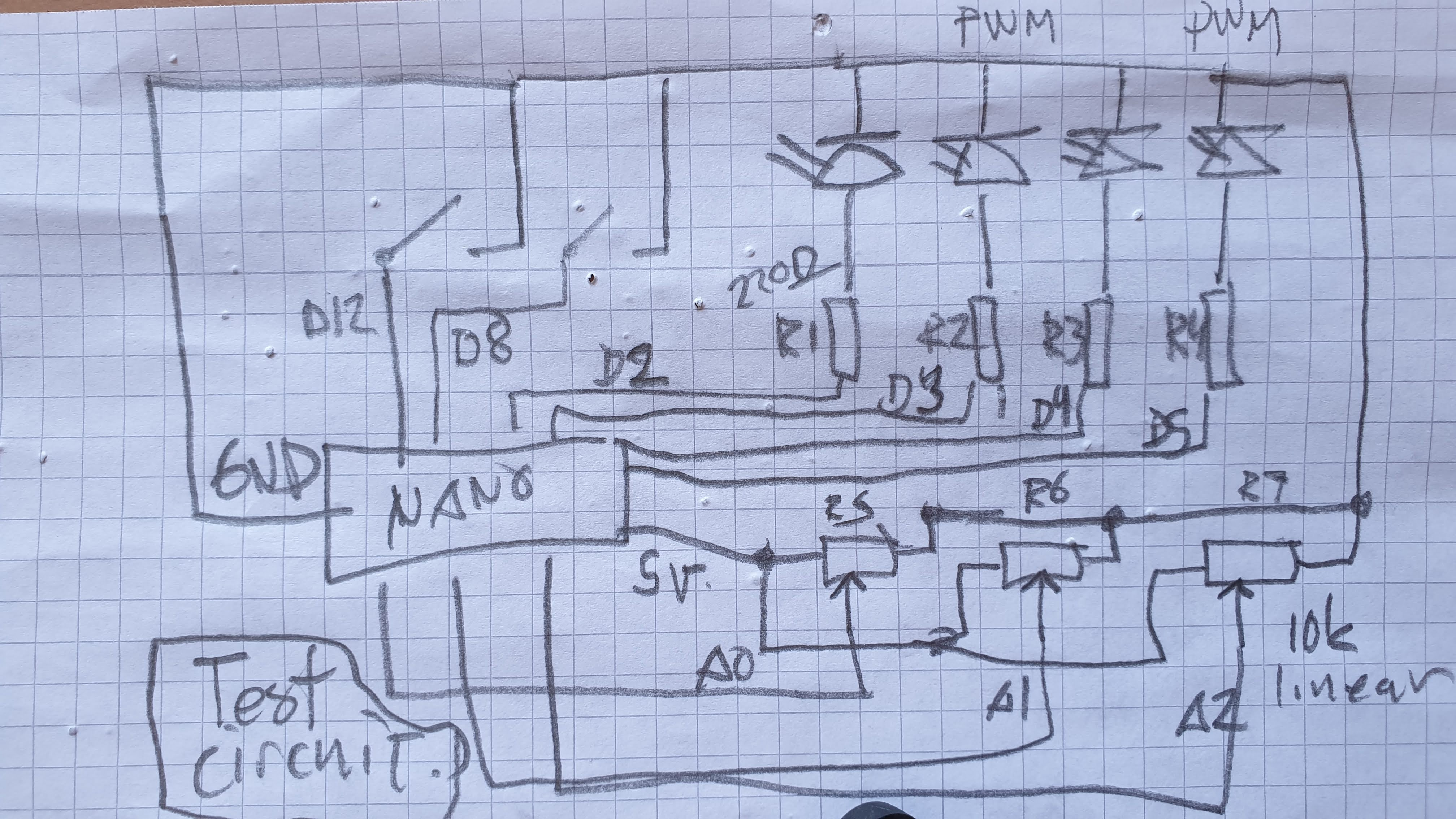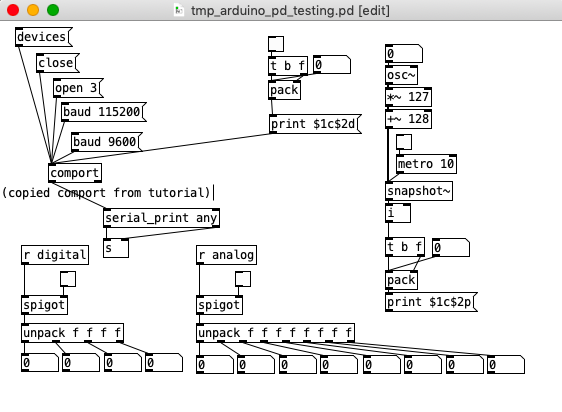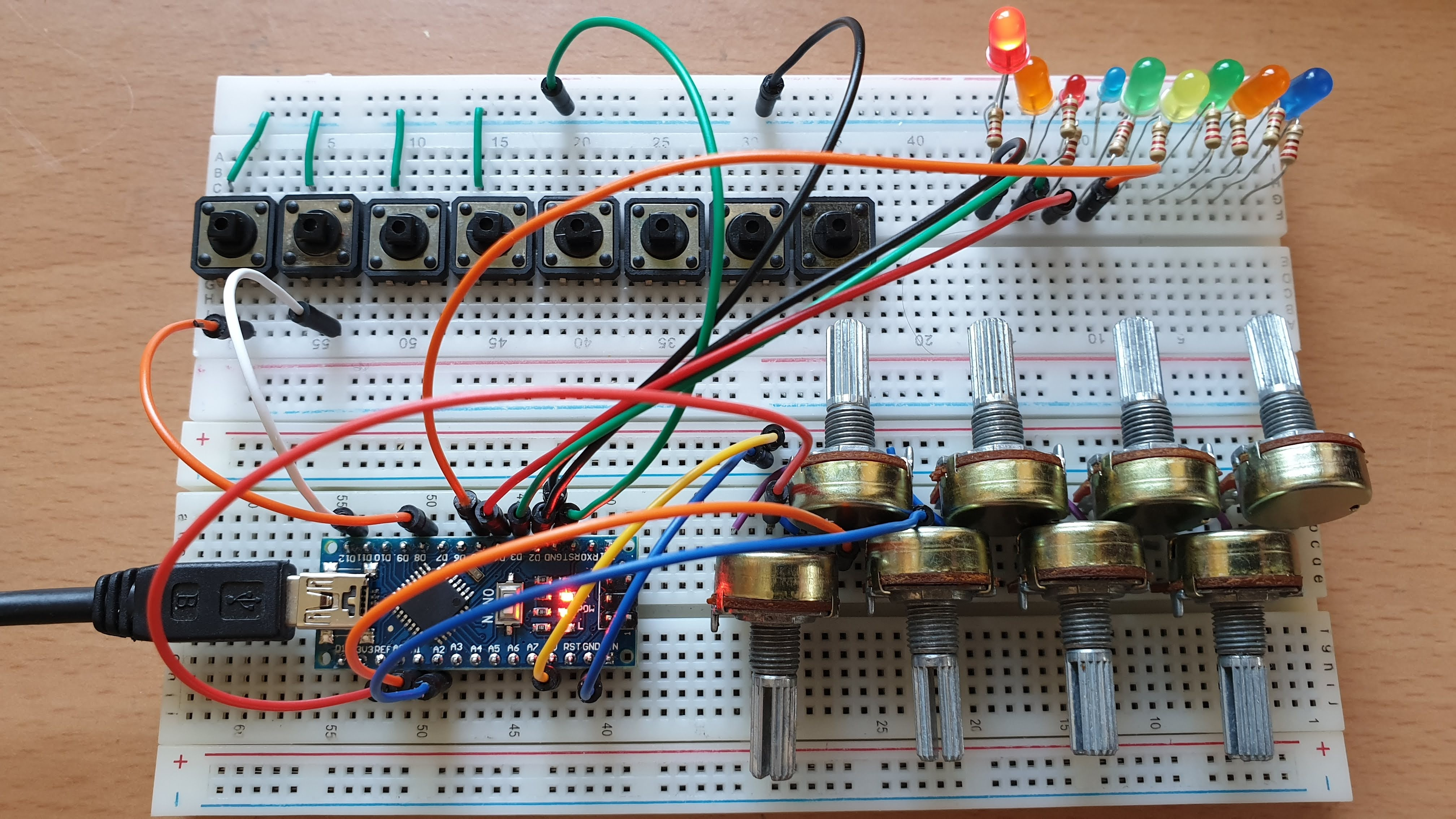``````int outPins = {2, 4, 7};
int pwmPins = {3, 5, 6, 9, 10, 11};

int channel = 0;

int inPins = {8, 12};
int analogPins = {0, 1, 2, 3, 4, 5, 6, 7};

// int debug_skip = 0;

void setup() {

for (int i = 0; i < 3; i++) {
pinMode(outPins[i], OUTPUT);
}
for (int i = 0; i < 6; i++) {
pinMode(pwmPins[i], OUTPUT);
}
for(int i = 0; i < 2; i++) {
pinMode(inPins[i], INPUT_PULLUP);
}

Serial.begin(115200);

}

void loop() {

//DO OUTPUTS

if (Serial.available()) {

static int temp;

if (isDigit(in)) temp = temp * 10 + in - '0';

//quote out this section to avoid crash

else if (in == 'c') {
channel = temp;
temp = 0;
}

else if (in == 'd') {
digitalWrite(outPins[channel], temp);
temp = 0;
}

else if (in == 'p') {
analogWrite(pwmPins[channel], temp);
temp = 0;
}

//end quote out here

}

// DO INPUTS

Serial.print("analog");

for(int i = 0; i < 8; i++){

unsigned int analogVal = analogRead (analogPins[i]);

Serial.print(" ");
Serial.print(analogVal);
}

Serial.println();

Serial.print("digital");

for(int i = 0; i < 2; i++) {

Serial.print(" ");
Serial.print(digitalVal);
}

Serial.println();

}
``````

If I do the following PureData will always freeze and requires force quit. Occasionally the whole OS becomes sluggish/freeze and need a reboot.

1. Have this code snippet in the Arduino sketch
``````    //quote out this section to avoid crash

else if (in == 'c') {
channel = temp;
temp = 0;
}

else if (in == 'd') {
digitalWrite(outPins[channel], temp);
temp = 0;
}

else if (in == 'p') {
analogWrite(pwmPins[channel], temp);
temp = 0;
}

//end quote out here
``````
1. Connect [print \$1c\$2p] -> [comport]

(I have also noted different behavior using [comport] from the tutorial by copy and paste compared to creating a comport object (cmd+1 and then typing). At times the latter did not work at all, but after reboot of the system both seems to work.)

Thanks for helping

Here is the patch from screenshot above:
tmp_arduino_pd_testing.pd

• Have you detected whether the crash happens with `else if (in == 'd')` or `else if (in == 'p')`?
In the Pd patch it's probably better to swap the \$1 and \$2 values in the messages. Have the channel set in a cold inlet and the value in a hot one and then write this message `print \$2c\$1v`
I see in the screenshot of the patch that the PWM message is not connected to [comport]. Is this for some particular reason? Maybe the 10ms interval for sending a PWM value to [comport] is a bit too fast. Did you try something slower, like 50ms?
Another thing would be to try and merge messages for digital and PWM. Since PWM happens automatically, you could append the digital pin message and send something like `print \$2c\$1p\$4c\$3d` where \$4 will be the digital pin channel and \$3 the value.

• @alexandros said:

Have you detected whether the crash happens with `else if (in == 'd')` or `else if (in == 'p')`?

It happens only in `else if (in == 'p')`. When I bypass that I can update snapshot~ by 1 ms (I assume that 'c' will still be sent by 1 ms intervals).

In the Pd patch it's probably better to swap the \$1 and \$2 values in the messages. Have the channel set in a cold inlet and the value in a hot one and then write this message `print \$2c\$1v`

Ok I did that.

I see in the screenshot of the patch that the PWM message is not connected to [comport]. Is this for some particular reason?

It is to make sure Pd does not crash/freeze until I want to try the code.

Maybe the 10ms interval for sending a PWM value to [comport] is a bit too fast. Did you try something slower, like 50ms?

That made it work. If I use six pwm pins the limit is 150 ms before crash.

Question 1: Could there something bad that happens around `else if (in == 'p')`? In another code snippet you used this format:

``````  while(Serial.available()){
if((inByte >= '0') && (inByte <= '9'))
temporary = 10 * temporary + inByte - '0';
else{
if(inByte == 'p'){
pwmLEDvalue = temporary;
temporary = 0;
}
else if(inByte == 'd'){
dspLEDstate = temporary;
temporary = 0;
}
}
analogWrite(pwmLED, pwmLEDvalue);
digitalWrite(dspLED, dspLEDstate);
}
``````

compared to this in the current sketch:

``````if (Serial.available()) {

static int temp;

if (isDigit(in)) temp = temp * 10 + in - '0';

else if (in == 'c') {
channel = temp;
temp = 0;
}

else if (in == 'd') {
digitalWrite(outPins[channel], temp);
temp = 0;
}

else if (in == 'p') {
analogWrite(pwmPins[channel], temp);
temp = 0;
}

}
``````

Question 2: Is this crash of Pd and the computer (macOS Mojave) expected behavior in a situation like this? If it is not, maybe it should be reported as a bug. It happens consistently.

Thoughts: Could there be a problem in [comport]? This crash behavior is identical with the problems I had when using Pdunio/Firmata.

• First of all, glad it finally worked!
Answer 1: The current code looks better than the one you posted above it (which is apparently something I've used, hopefully in my tutorial and not the book...), because analogWrite() and digitalWrite() are only called when needed. In the first code snippet both functions are called whenever there's data in the serial line, which is overhead.
Answer 2: I don't really have an answer to this. You can send an email to Pd's mailing list where Martin Peach, the developer of [comport] is active. It does sound strange that your computer crashes, as a longer delay just gives time to the Arduino to do the PWM stuff, but that has to do with the Arduino. Maybe there's something strange going on when the Arduino doesn't have enough time to do what it has to do, but I really don't know.

• @alexandros said:

Answer 1: The current code looks better than the one you posted above it (which is apparently something I've used, hopefully in my tutorial and not the book...),

It is from the tutorial and I believe it was fine in a stepwise educational context. (Why I posted it was because the variables inside the else if() are treated differently. Wild guess that it could be a problem.)

Thank you so much for that you took time to help me on this. However, this problem does not exist in the old tutorial code(!).

Look here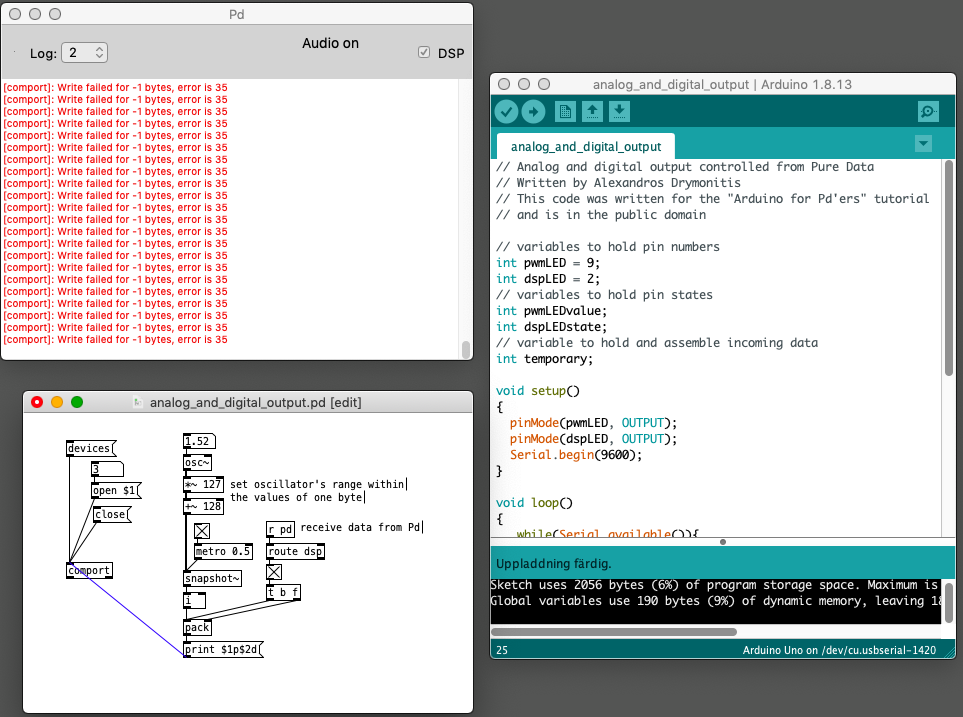I send two updates per millisecond and still no crash. The Pd console reports errors. Pd still works as normal. This is the codes (Arduino and Pd) from your tutorial.

When using the new codes (both Ardunio sketch and Pd patch) Pd crash/freeze and there is no report in the pd console.

Posts 40 | Views 10734
Internal error.

Oops! Looks like something went wrong!